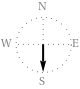Search IntMath
Close

450+ Math Lessons written by Math Professors and Teachers

5 Million+ Students Helped Each Year

1200+ Articles Written by Math Educators and Enthusiasts

Simplifying and Teaching Math for Over 23 Years

# 180 Degrees to Radians in Geometry## What is 180 Degrees?

In geometry, 180 degrees is the angle formed when two straight lines intersect at their endpoints. This angle is also known as a straight angle or a flat angle. It is most commonly represented by the symbol ?180°.

A radian is a unit of angular measure. It is defined as the angle that is formed when you draw a radius of a circle and trace an arc that is equal in length to the radius. A radian is equal to the ratio of the arc length (s) to the radius (r) of a circle, or s/r. This translates to the equation of 1 radian = 57.2958 degrees.

The conversion from radians to degrees is simple. All you need to do is multiply the number of radians by 57.2958 to get the equivalent number of degrees. For example, if you wanted to convert 2 radians to degrees, you would multiply 2 by 57.2958 to get 114.5916 degrees.

Converting from degrees to radians is just as easy. Just divide the number of degrees by 57.2958 to get the equivalent number of radians. For example, if you wanted to convert 180 degrees to radians, you would divide 180 by 57.2958 to get 3.1416 radians.

## Angular Conversion in Geometry and Trigonometry

Angular conversions are important in geometry and trigonometry because they are used to calculate the lengths of sides and angles in various shapes. The radian measure is often used in trigonometric functions, such as sine and cosine, to convert angles between degrees and radians.

## Practice Problems

Let's practice converting between degrees and radians.

• 1. Convert 45 degrees to radians: 45 degrees divided by 57.2958 equals 0.7854 radians
• 2. Convert 3.1416 radians to degrees: 3.1416 radians multiplied by 57.2958 equals 180 degrees
• 3. Convert 135 degrees to radians: 135 divided by 57.2958 equals 2.3562 radians
• 4. Convert 6.283 radians to degrees: 6.283 radians multiplied by 57.2958 equals 360 degrees
• 5. Convert 30 degrees to radians: 30 divided by 57.2958 equals 0.5236 radians
• 6. Convert 2.3562 radians to degrees: 2.3562 radians multiplied by 57.2958 equals 135 degrees

## Summary

In this article, we discussed 180 degrees to radians in geometry. We learned that 180 degrees is an angle formed when two straight lines intersect at their endpoints. We also learned that a radian is a unit of angular measure and is equal to the ratio of the arc length to the radius of a circle. We then discussed how to convert from radians to degrees and from degrees to radians. Finally, we practiced these conversions with some practice problems.

## FAQ

### Why is 180 degrees equal to pi radians?

180 degrees is equal to pi radians because there are 2p radians in a full circle, which is equal to 360 degrees. Since 180 degrees is half of 360 degrees, it is equal to half of 2p radians, which is equal to pi radians.

### How do we convert degrees to radians?

To convert degrees to radians, you can use the formula radians = (degrees x p) / 180. This formula can be used to convert any angle in degrees to its equivalent angle in radians.

### How do you find radians in geometry?

Radians can be found in geometry by using the formula radians = (degrees x p) / 180. This formula can be used to convert any angle in degrees to its equivalent angle in radians. Additionally, radians can be found in the context of a circle, where one full rotation is equal to 2p radians.